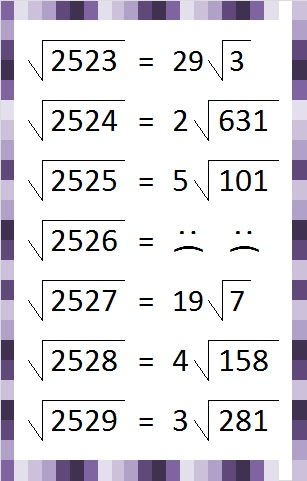# 281 and More Consecutive Square Roots

• 281 is a prime number.
• Prime factorization: 281 is prime.
• The exponent of prime number 281 is 1. Adding 1 to that exponent we get (1 + 1) = 2. Therefore 281 has exactly 2 factors.
• Factors of 281: 1, 281
• Factor pairs: 281 = 1 x 281
• 281 has no square factors that allow its square root to be simplified. √281 ≈ 16.763How do we know that 281 is a prime number? If 281 were not a prime number, then it would be divisible by at least one prime number less than or equal to √281 ≈ 16.763. Since 281 cannot be divided evenly by 2, 3, 5, 7, 11, or 13, we know that 281 is a prime number.

So far I have posted about one set of four and two sets of five consecutive reducible square roots. Sets of three consecutive reducible square roots are fairly common so I’ve ignored most of them. These consecutive square roots couldn’t be ignored:The final square root features today’s prime number 281. Here are the prime factorizations and number of factors of each of these numbers:Six is a popular number when counting the number of factors.

This site uses Akismet to reduce spam. Learn how your comment data is processed.